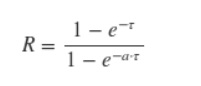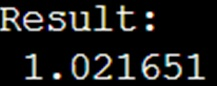# How to solve an equation using a NumPy numerical solver?

Given an equation, we have to solve it using Python's numerical solver in NumPy.
Submitted by Pranit Sharma, on March 13, 2023

## Solving an equation using a NumPy numerical solver

Suppose that we are given a mathematical equation:

R - ((1.0 - np.exp(-tau))/(1.0 - np.exp(-a*tau))) = 0, and we need to solve this equation for tau.

The above can be mathematically represented as:We can solve this equation using scipy. SciPy's fsolve() function searches for a point at which a given expression equals zero (a "zero" or "root" of the expression). We just need to provide fsolve() with an initial guess that is "near" your desired solution. A good way to find such an initial guess is to just plot the expression and look for the zero crossing.

Let us understand with the help of an example,

## Python code to solve an equation using a NumPy numerical solver

```# Import numpy
import numpy as np

# Import fsolve
from scipy.optimize import fsolve

# Defininig the expression
a = 0.5
R = 1.6
fun = lambda tau : R - ((1.0 - np.exp(-tau))/(1.0 - np.exp(-a*tau)))

# tau
tau = np.linspace(-0.5, 1.5, 201)

# Initial guess
guess = 0.5

# Solving the equation
res = fsolve(fun, guess)

print("Result:\n %f" % res)
```

### OutputLanguages: » C » C++ » C++ STL » Java » Data Structure » C#.Net » Android » Kotlin » SQL
Web Technologies: » PHP » Python » JavaScript » CSS » Ajax » Node.js » Web programming/HTML
Solved programs: » C » C++ » DS » Java » C#
Aptitude que. & ans.: » C » C++ » Java » DBMS
Interview que. & ans.: » C » Embedded C » Java » SEO » HR
CS Subjects: » CS Basics » O.S. » Networks » DBMS » Embedded Systems » Cloud Computing
» Machine learning » CS Organizations » Linux » DOS
More: » Articles » Puzzles » News/Updates